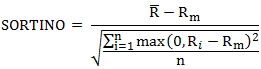# SQL Server Sortino ratio

SORTINO2

Updated: 04 April 2015

Use the aggregate function SORTINO2 to calculate the Sortino ratio based upon price data. The Sortino ratio is calculated as the mean difference of the returns (R) and the minimum acceptable return (Rm) divided by the downside deviation.Where

 R¯ = the average of the returns Rm = the minimum acceptable return Ri = the ith return n = When @Full = 'TRUE' then the number of rows passed into the function minus 1; else the number of rows where max(0, Ri – Rm) < 0.

Syntax
SELECT [wct].[SORTINO2](
<@PDate, datetime,>
<@PValue, float,>
,<@Mar, float,>
,<@Full, bit,>)

Arguments
@PDate
the date associated with the price or valuation. @PDate is an expression of type datetime or of a type that can be implicitly converted to datetime.
@PValue
the price or value for the @PDate. @PDate is an expression of type float or of a type that can be implicitly converted to float.
@Mar
the minimum acceptable return. @Mar is an expression of type float or of a type that can be implicitly converted to float.
@Full
a bit value which defines how to calculate the downside deviation. When @Full = 'TRUE' the downside deviation is calculated with n equal to the number of rows passed into the function minus 1. When @Full = 'FALSE' n is equal to the number of rows where max(0,R – Rm) < 0.

Return Type
float

Remarks
·         If there are no negative returns, then SORTINO2 is NULL.
·         @Mar must be the same for all rows in the GROUP BY.
·         @Full must be the same for all rows in the GROUP BY.
·         @Full defaults to'FALSE'.
·         Available in XLeratorDB / financial 2008 only

Examples
In this example, we have 13 monthly portfolio valuation, enabling us to calculate the monthly returns for 12 months. Our minimum acceptable return is 10% per year, which we turn into a monthly return using the formula 1.101/12-1. The downside deviation is calculated with n = 12.
DECLARE @MAR as float = POWER(1.1e+00,1E+00/12e+00)-1

SELECT
wct.SORTINO2(
mth
,pr
,@MAR
,'True'
) as Sortino
FROM (VALUES
('2013-12-31',1000000.00)
,('2014-01-31',1008900.00)
,('2014-02-28',1010110.68)
,('2014-03-31',1008090.46)
,('2014-04-30',1018171.36)
,('2014-05-31',1017967.73)
,('2014-06-30',1038327.08)
,('2014-07-31',1069476.90)
,('2014-08-31',1080171.66)
,('2014-09-30',1076931.15)
,('2014-10-31',1087700.46)
,('2014-11-30',1098795.01)
,('2014-12-31',1087807.06)
)n(mth,pr)
This produces the following result.

Using the same data as above, we change @Full = 'FALSE' and the downside deviation is calculated with n = 5.

DECLARE @MAR as float = POWER(1.1e+00,1E+00/12e+00)-1

SELECT
wct.SORTINO2(
mth
,pr
,@MAR
,'False'
) as Sortino
FROM (VALUES
('2013-12-31',1000000.00)
,('2014-01-31',1008900.00)
,('2014-02-28',1010110.68)
,('2014-03-31',1008090.46)
,('2014-04-30',1018171.36)
,('2014-05-31',1017967.73)
,('2014-06-30',1038327.08)
,('2014-07-31',1069476.90)
,('2014-08-31',1080171.66)
,('2014-09-30',1076931.15)
,('2014-10-31',1087700.46)
,('2014-11-30',1098795.01)
,('2014-12-31',1087807.06)
)n(mth,pr)
This produces the following result.Copyright 2008-2023 Westclintech LLC         Privacy Policy        Terms of Service